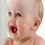## Friday, July 23, 2010

### Branch and Bound Algorithem.

ALGORITHM: Branch - and - Bound search

1. Place the start node of zero path length on the queue.

2. Until the queue is empty or a goal node has been found do the following:

(i) Determine if the s first path in the queue contains a good node.

(ii) If the first path contains a goal node exit with success.

(iii) If the first path does not contain a good node remove the path from the queue and form new paths by extending the removed paths by on step.

(iv) Compute the cost of the new paths and add them to the queue.

(v) Sort the paths on the queue with lowest-cost path in front.

3. otherwise exit with failure.

Now let us see how the branch - and -bound search could be used to find the shortest solution to the water- jug problem. Unfortunately although this algorithm is more efficient and simple. It require exponential time. The exact amount of time it saves for particular problem depends on the order in which the paths are explored. And also in this problem, there is a possibility that the algorithm enters into an indefinite loop. For example , consider the following configuration generated in the process of generating a path.

1.2.3.